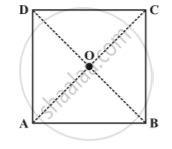Share

# Some Special Parallelograms - Concept of a Square

#### description

• Property: The diagonals of a square are perpendicular bisectors of each other.

#### notes

A square is a rectangle with equal sides. This means a square has all the properties of a rectangle with an additional requirement that all the sides have equal length.
In a square the diagonals.
(i) bisect one another (square being a parallelogram)
(ii) are of equal length (square being a rectangle) and
(iii) are perpendicular to one another.

Property: The diagonals of a square are perpendicular bisectors of each other.ABCD is a square whose diagonals meet at O .
OA = OC   (Since the square is a parallelogram)
By SSS congruency condition, we now see that
∆ AOD ≅ ∆ COD
Therefore, m∠AOD = m∠COD
These angles being a linear pair, each is right angle.

### Shaalaa.com

Property: The diagonals of a square are perpendicular bisectors of each other. [00:08:13]
S
0%

S